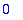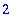Error: (in limit) invalid directional argument

The limit command has an optional third argument, which allows the calculation of various types of limits, e.g. one-sided limits. The allowed values for this argument are left , right , real and complex . Anything else produces this error message.

One way this error sometimes arises is that someone uses left or right as a variable and assigns it a value. It seems to work at first: these are not protected names. But when you try to evaluate a definite integral, this error appears. The reason is that Maple's code for definite integrals calls limit with the options left and right to evaluate the antiderivative at the endpoints of its interval. If left or right has been assigned a value, this will not work.

Examples:

> limit(2^(1/x), x=0, left);> limit(2^(1/x), x=0, LEFT);

Error, (in limit) invalid directional argument

Assigning a value to left causes errors:

> left:= 4:

> limit(2^(1/x), x=0, left);

Error, (in limit) invalid directional argument

> int(x, x=0 .. 2);

Error, (in limit) invalid directional argument

Unassigning left fixes the problem.

> left:= 'left':

> int(x, x=0 .. 2);